# Maharashtra Board 12th Commerce Maths Solutions Chapter 3 Linear Regression Ex 3.3

Balbharati Maharashtra State Board 12th Commerce Maths Digest Pdf Chapter 3 Linear Regression Ex 3.3 Questions and Answers.

## Maharashtra State Board 12th Commerce Maths Solutions Chapter 3 Linear Regression Ex 3.3

Question 1.
From the two regression equations find r, $$\bar{x}$$ and $$\bar{y}$$.
4y = 9x + 15 and 25x = 4y + 17
Solution:
Given 4y = 9x + 15 and 25x = 4y + 17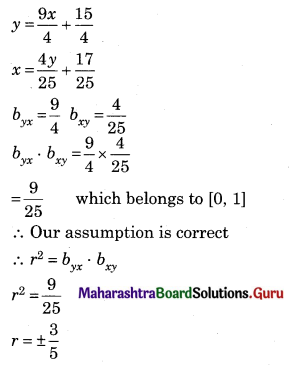Since byx and bxy are positive.
∴ r = $$\frac{3}{5}$$ = 0.6
($$\bar{x}$$, $$\bar{y}$$) is the point of intersection of the regression lines
9x – 4y = -15 …….(i)
25x – 4y = 17 ……….(ii)
-16x = -32
x = 2
∴ $$\bar{x}$$ = 2
Substituting x = 2 in equation (i)
9(2) – 4y = -15
18 + 15 = 4y
33 = 4y
y = 33/4 = 8.25
∴ $$\bar{y}$$ = 8.25Question 2.
In a partially destroyed laboratory record of an analysis of regression data, the following data are legible:
Variance of X = 9
Regression equations:
8x – 10y + 66 = 0 And 40x – 18y = 214.
Find on the basis of the above information
(i) The mean values of X and Y.
(ii) Correlation coefficient between X and Y.
(iii) Standard deviation of Y.
Solution:
Given, $$\sigma_{x}{ }^{2}=9, \sigma_{x}=3$$
(i) ($$\bar{x}$$, $$\bar{y}$$) is the point of intersection of the regression lines
40x – 50y = -330 …….(i)
40x – 50y = +214 ………(ii)
-32y = -544
y = 17
∴ $$\bar{y}$$ = 17
8x – 10(17) + 66 = 0
8x = 104
x = 13
∴ $$\bar{x}$$ = 13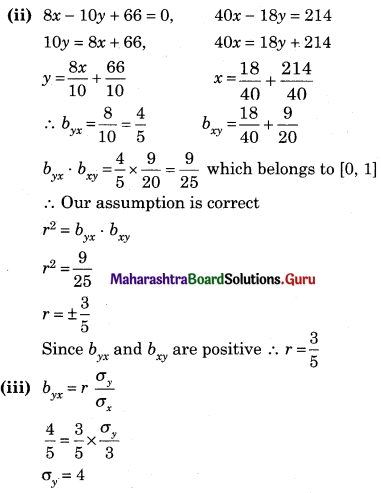Question 3.
For 50 students of a class, the regression equation of marks in statistics (X) on the marks in Accountancy (Y) is 3y – 5x + 180 = 0. The mean marks in accountancy is 44 and the variance of marks in statistics $$\left(\frac{9}{16}\right)^{t h}$$ of the variance of marks in accountancy. Find the mean in statistics and the correlation coefficient between marks in two subjects.
Solution:
Given, n = 50, $$\bar{y}$$ = 44
$$\sigma_{x}^{2}=\frac{9}{16} \sigma_{y}^{2}$$
∴ $$\frac{\sigma_{x}}{\sigma_{x}}=\frac{3}{4}$$
Since ($$\bar{x}$$, $$\bar{y}$$) is the point intersection of the regression line.
∴ ($$\bar{x}$$, $$\bar{y}$$) satisfies the regression equation.
3$$\bar{y}$$ – 5$$\bar{x}$$ + 180 = 0
3(44) – 5$$\bar{x}$$ + 180 = 0
∴ 5$$\bar{x}$$ = 132 + 180
$$\bar{x}$$ = $$\frac{312}{5}$$ = 62.4
∴ Mean marks in statistics is 62.4
Regression equation of X on Y is 3y – 5x + 180 = 0
∴ 5x = 3y + 180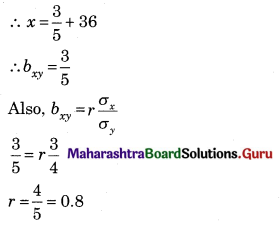Question 4.
For bivariate data, the regression coefficient of Y on X is 0.4 and the regression coefficient of X on Y is 0.9. Find the value of the variance of Y if the variance of X is 9.
Solution: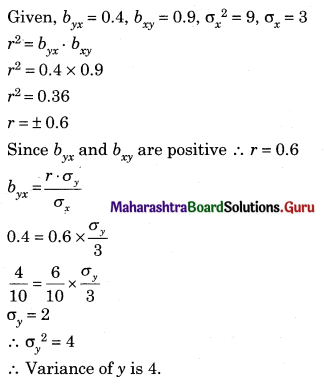Question 5.
The equation of two regression lines are 2x + 3y – 6 = 0 and 3x + 2y – 12 = 0
Find (i) Correlation coefficient (ii) $$\frac{\sigma_{x}}{\sigma_{y}}$$
Solution: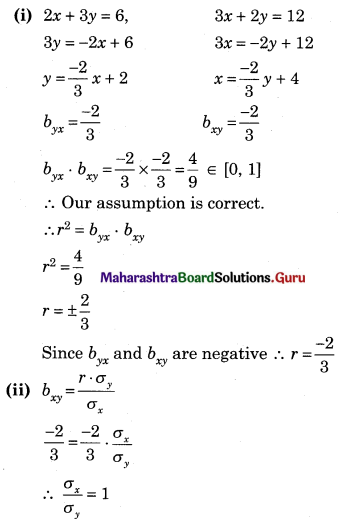Question 6.
For a bivariate data $$\bar{x}$$ = 53, $$\bar{y}$$ = 28, byx =-1.5 and bxy = -0.2. Estimate Y when X = 50.
Solution:
Regression equation of Y on X is
(Y – $$\bar{y}$$) = byx (X – $$\bar{x}$$)
(Y – 28) = -1.5(50 – 53)
Y – 28 = -1.5(-3)
Y – 28 = 4.5
Y = 32.5Question 7.
The equation of two regression lines are x – 4y = 5 and 16y – x = 64. Find means of X and Y. Also, find the correlation coefficient between X and Y.
Solution:
Since ($$\bar{x}$$, $$\bar{y}$$) is the point of intersection of the regression lines.
x – 4y = 5 …..(i)
-x + 16y = 64 …….(ii)
12y = 69
y = 5.75
Substituting y = 5.75 in equation (i)
x – 4(5.75) = 5
x – 23 = 5
x = 28
∴ $$\bar{x}$$ = 28, $$\bar{y}$$ = 5.75
x – 4y = 5
x = 4y + 5
∴ bxy = 4
16y – x = 64
16y = x + 64
y = $$\frac{1}{16}$$x + 4
byx = $$\frac{1}{16}$$
byx . bxy = $$\frac{1}{16}$$ × 4 = $$\frac{1}{4}$$ ∈ [0, 1]
∴ Our assumption is correct
∴ r2 = byx . bxy
r2 = $$\frac{1}{4}$$
r = ±$$\frac{1}{2}$$
Since byx and bxy are positive,
∴ r = $$\frac{1}{2}$$ = 0.5

Question 8.
In partially destroyed record, the following data are available variance of X = 25. Regression equation of Y on X is 5y – x = 22 and Regression equation of X on Y is 64x – 45y = 22 Find
(i) Mean values of X and Y.
(ii) Standard deviation of Y.
(iii) Coefficient of correlation between X and Y.
Solution:
Given $$\sigma_{x}^{2}$$ = 25, ∴ σx = 5
(i) Since ($$\bar{x}$$, $$\bar{y}$$) is the point of intersection of regression lines
-x + 5y = 22 …….(i)
64x – 45y = 22 ………..(ii)
equation (i) becomes
-9x + 45y = 198
64y – 45y = 22
55x = 220
x = 4
Substituting x = 4 in equation (i)
-4 + 5y = 22
5y = 26
∴ y = 5.2
∴ $$\bar{x}$$ = 4, $$\bar{y}$$ = 5.2
Regression equation of X on Y is
64x – 45y – 22
64x = 45y + 22
x = $$\frac{45}{64} y+\frac{22}{64}$$
bxy = $$\frac{45}{64}$$
(ii) Regression equation of Y on X is
5y – x = 22
5y = x + 22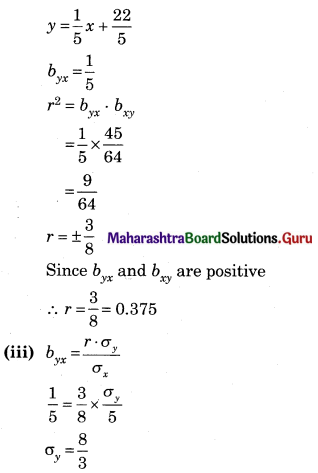Question 9.
If the two regression lines for a bivariate data are 2x = y + 15 (x on y) and 4y – 3x + 25 (y on x) find
(i) $$\bar{x}$$
(ii) $$\bar{y}$$
(iii) byx
(iv) bxy
(v) r [Given √0.375 = 0.61]
Solution:
Since ($$\bar{x}$$, $$\bar{y}$$) is the point of intersection of the regression line
2x = y + 15
4y = 3x + 25
2x – y = 15 …….(i)
3x – 4y = -25 ……..(ii)
Multiplying equation (i) by 4
8x – 4y = 60
3x – 4y = -25
on Subtracting,
5x = 85
∴ x = 17
Substituting x in equation (i)
2(17) – y = 15
34 – 15 = y
∴ y = 15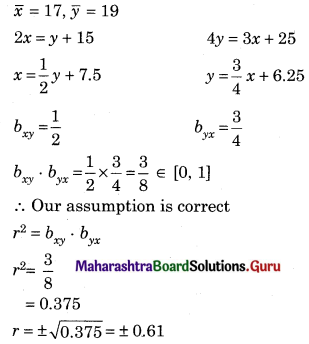Since byx and bxy are positive, ∴ r = 0.61Question 10.
The two regression equation are 5x – 6y + 90 = 0 and 15x – 8y – 130 = 0. Find $$\bar{x}$$, $$\bar{y}$$, r.
Solution:
Since ($$\bar{x}$$, $$\bar{y}$$) is the point of intersection of the regression lines
5x – 6y + 90 = 0 ……(i)
15x – 8y – 130 = 0
15x – 18y + 270 = 0
15x – 8y – 130 = 0
on subtracting,
-10y + 400 = 0
y = 40
Substituting y = 40 in equation (i)
5x – 6(40) + 90 = 0
5x = 150
x = 30
∴ $$\bar{x}$$ = 30, $$\bar{y}$$ = 40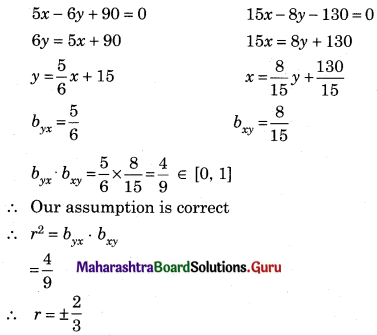Since byx and bxy are positive
∴ r = $$\frac{2}{3}$$

Question 11.
Two lines of regression are 10x + 3y – 62 = 0 and 6x + 5y – 50 = 0 Identify the regression equation equation of x on y. Hence find $$\bar{x}$$, $$\bar{y}$$, and r.
Solution: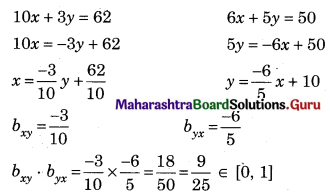∴ Our assumption is correct.
∴ Regression equation of X on Y is 10x + 3y – 62 = 0
r2 = byx . bxy
r2 = $$\frac{9}{25}$$
r = ±$$\frac{3}{5}$$
Since, byx and bxy are negative, r = –$$\frac{3}{5}$$ = -0.6
Also ($$\bar{x}$$, $$\bar{y}$$) is the point of intersection of the regression lines
50x + 15y = 310
18x + 15y = 150
on subtracting
32x = 160
x = 5
Substituting x = 5 in 10x + 3y = 62
10(5) + 3y = 62
3y = 12
∴ y = 4
∴ $$\bar{x}$$ = 5, $$\bar{y}$$ = 4

Question 12.
For certain X and Y series, which are correlated the two lines of regression are 10y = 3x + 170 and 5x + 70 = 6y. Find the correlation coefficient between them. Find the mean values of X and Y.
Solution: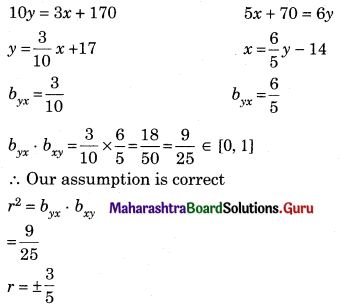Since byx and bxy are positive,
r = $$\frac{3}{5}$$ = 0.6
Since, ($$\bar{x}$$, $$\bar{y}$$) is the point of intersection of the regression lines
3x – 10y = -170 …….(i)
5x – 6y = -70 ………(ii)
9x – 30y = -510
25x – 30y = -350
on subtracting
-16x = -160
x = 10
Substituting x = 10 in equation (i)
3(10) – 10y = -170
30 + 170 = 10y
200 = 10y
y = 20
∴ $$\bar{x}$$ = 10, $$\bar{y}$$ = 20Question 13.
Regression equation of two series are 2x – y – 15 = 0 and 4y + 25 = 0 and 3x- 4y + 25 = 0. Find $$\bar{x}$$, $$\bar{y}$$ and regression coefficients, Also find coefficients of correlation. [Given √0.375 = 0.61]
Solution:
Since ($$\bar{x}$$, $$\bar{y}$$) is the point of intersection of the regression line
2x = y + 15
4y = 3x + 25
2x – y = 15 ……(i)
3x – 4y = -15 ……..(ii)
Multiply equation (i) by 4
8x – 4y = 60
3x – 4y = -25
on subtracting,
5x = 85
x = 17
Substituting x in equation (i)
2(17) – y = 15
34 – 15 = y
y = 15
∴ $$\bar{x}$$ = 17, $$\bar{y}$$ = 19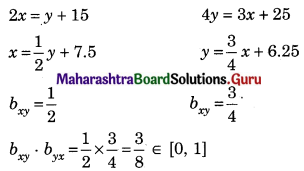∴ Our assumption is correct
r2 = bxy . byx
r2 = $$\frac{3}{8}$$ = 0.375
r = ±√o.375 = ±0.61
Since, byx and bxy are positive, ∴ r = 0.61

Question 14.
The two regression lines between height (X) in includes and weight (Y) in kgs of girls are 4y – 15x + 500 = 0 and 20x – 3y – 900 = 0. Find the mean height and weight of the group. Also, estimate the weight of a girl whose height is 70 inches.
Solution:
Since ($$\bar{x}$$, $$\bar{y}$$) is the point intersection of the regression lines
15x – 4y = 500 ……(i)
20x – 3y = 900 …….(ii)
60x – 16y – 2000
60x – 9y = 2700
on subtracting,
-7y = -700
y = 100
Substituting y = 100 in equation (i)
15x – 4(100) = 500
15x = 900
x = 60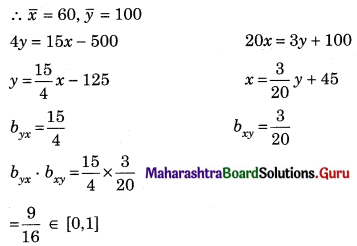∴ Our assumption is correct
∴ Regression equation of Y on X is
Y = $$\frac{15}{4}$$x – 125
When x = 70
Y = $$\frac{15}{4}$$ × 70 = -125
= 262.5 – 125
= 137.5 kg